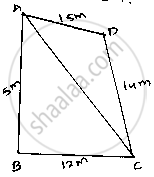# The Sides of a Quadrilateral, Taken in Order Are 5, 12, 14 and 15 Meters Respectively, and the Angle Contained by the First Two Sides is a Right Angle. Find Its Are - Mathematics

The sides of a quadrilateral, taken in order are 5, 12, 14 and 15 meters respectively, and the angle contained by the first two sides is a right angle. Find its are

#### Solution

Given that sides of quadrilateral are AB = 5 m, BC = 12 m, CD = 14 m and DA = 15 m
AB = 5m, BC = 12m, CD = 14 m and DA = 15 m
Join AC

Area of ΔABC = 1/2×𝐴𝐵×𝐵𝐶       [∵𝐴𝑟𝑒𝑎 𝑜𝑓 Δ𝑙𝑒=1/2(3𝑥+1)]

= 1/2×5×12

= 30 cm2

In ΔABC By applying Pythagoras theorem.

AC^2=AB^2+BC^2

⇒AC=sqrt(5^2+12^2)

⇒sqrt(25+144)

⇒sqrt169=13m

𝑁𝑜𝑤 𝑖𝑛 Δ𝐴𝐷𝐶
Let 2s be the perimeter∴ 2s = (AD + DC + AC)

⇒ S = 1/2(15+14+13)=1/2×42=21𝑚

By using Heron’s formula

∴ Area of ΔADC = sqrt(S(S-AD)(S-DC)(S-AC))

=sqrt(21(21-15)(21-14)(21-13))

sqrt(21xx6xx7xx8)

∴𝐴𝑟𝑒𝑎 𝑜𝑓 𝑞𝑢𝑎𝑑𝑟𝑖𝑙𝑎𝑡𝑒𝑟𝑎𝑙 𝐴𝐵𝐶𝐷=𝑎𝑟𝑒𝑎 𝑜𝑓 (Δ𝐴𝐵𝐶)+𝐴𝑟𝑒𝑎 𝑜𝑓 (Δ𝐴𝐷𝐶) = 30 + 84 = 114 m^2

Concept: Application of Heron’s Formula in Finding Areas of Quadrilaterals
Is there an error in this question or solution?

#### APPEARS IN

RD Sharma Mathematics for Class 9
Chapter 17 Heron’s Formula
Exercise 17.2 | Q 3 | Page 19

Share